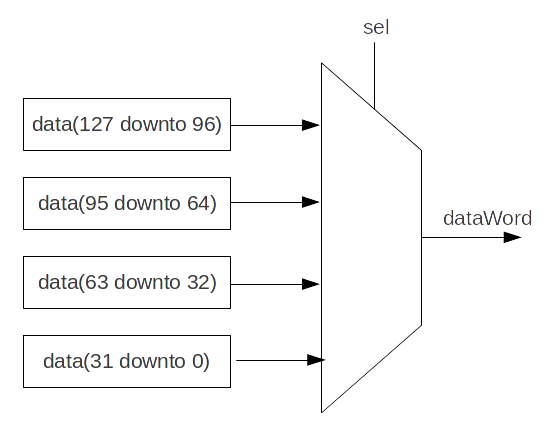You're reading an old version of this documentation.
For the latest stable release version, please have a look at master.

# When/Switch/Mux

## When

As VHDL and Verilog, signals can be conditionally assigned when a special condition is met.

```when(cond1){
//execute when      cond1 is true
}.elsewhen(cond2){
//execute when (not cond1) and cond2
}.otherwise{
//execute when (not cond1) and (not cond2)
}
```

## Switch

As VHDL and Verilog, signals can be conditionally assigned when a signal has a defined value.

```switch(x){
is(value1){
//execute when x === value1
}
is(value2){
//execute when x === value2
}
default{
//execute if none of precedent condition meet
}
}
```

## Local declaration

It’s possible to define new signals into a when/switch statement.

```val x,y = UInt(4 bits)
val a,b = UInt(4 bits)

when(cond){
val tmp = a + b
x := tmp
y := tmp + 1
} otherwise {
x := 0
y := 0
}
```

Note

SpinalHDL check that signals defined into a scope are only assigned inside this one.

## Mux

If you just need a Mux with a Bool selection signal, there is two equivalent syntaxes :

Syntax

Return

Description

Mux(cond, whenTrue, whenFalse)

T

Return `whenTrue` when `cond` is True, `whenFalse` otherwise

cond ? whenTrue | whenFalse

T

Return `whenTrue` when `cond` is True, `whenFalse` otherwise

```val cond = Bool
val whenTrue, whenFalse = UInt(8 bits)
val muxOutput  = Mux(cond, whenTrue, whenFalse)
val muxOutput2 = cond ? whenTrue | whenFalse
```

## Bitwise selection

A bitwise selection looks like the VHDL `when` syntax.

### Example

```val bitwiseSelect = UInt(2 bits)
val bitwiseResult = bitwiseSelect.mux(
0 -> (io.src0 & io.src1),
1 -> (io.src0 | io.src1),
2 -> (io.src0 ^ io.src1),
default -> (io.src0)
)
```

Also, if all possible values are covered in your mux, you can omit the default value :

```val bitwiseSelect = UInt(2 bits)
val bitwiseResult = bitwiseSelect.mux(
0 -> (io.src0 & io.src1),
1 -> (io.src0 | io.src1),
2 -> (io.src0 ^ io.src1),
3 -> (io.src0)
)
```

`muxLists(...)` is another bitwise selection which take as input a sequence of tuple. Below an example of dividing a Bits of 128 bits into 32 bits :```val sel  = UInt(2 bits)
val data = Bits(128 bits)

val dataWord = sel.muxList(for(index <- 0 until 4) yield (index, data(index*32+32-1 downto index*32)))

// This example can be written shorter.
val dataWord = data.subdivideIn(32 bits)(sel)
```